# How can I tell if Statistics are created by the system

Column statistics are used by the optimizer to help create the best possible execution plan for a query.  There are a few ways these statistics get created.  The first method is when an index is created, which are updated when the index is optimized. The second method of creating statistics is by using the CREATE STATISTICS statement.  This statement allows the DBA to create statistics on a column that doesn’t have an index on it and allows the creator to provide a name of the statistics object.  The last method is to let SQL Server create the statistics when the column is read.

Using the AdventureWorks2014 database, if you run the code below you will see that there are not any statistics on the MakeFlag column in the Production.Project table.

SELECT OBJECT_NAME(object_id) AS [ObjectName]
,[name] AS [StatisticName]
,STATS_DATE([object_id], [stats_id]) AS [StatisticUpdateDate]
FROM sys.stats
WHERE OBJECT_NAME(object_id) = ‘Product’

I get the result set below.  But in looking at it, how can I tell that there really isn’t statistics on the MakeFlag column?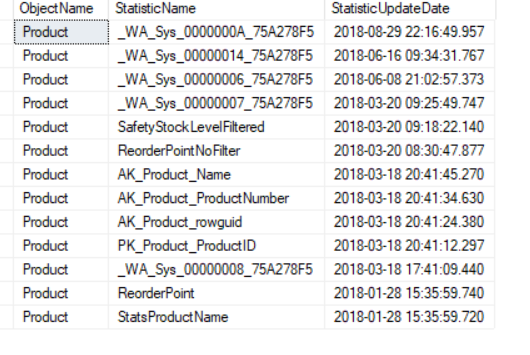You can run the statement below that will list all the columns that have statistics.  This statement can be found at here on Microsoft’s web site.

SELECT s.name AS statistics_name
,c.name AS column_name
,sc.stats_column_id
FROM sys.stats AS s
INNER JOIN sys.stats_columns AS sc
ON s.object_id = sc.object_id AND s.stats_id = sc.stats_id
INNER JOIN sys.columns AS c
ON sc.object_id = c.object_id AND c.column_id = sc.column_id
WHERE s.object_id = OBJECT_ID(‘Production.product’);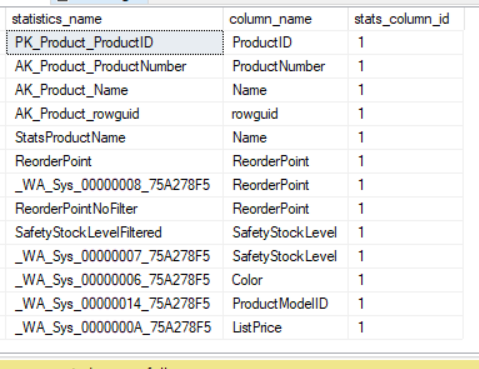Now that I have been able to check the columns, what are the objects that have the funny names?  Those are system generated statistics.  Let’s break down the name.

Using this name as an example: _WA_Sys_00000007_75A278F5

The WA really just stands for Washington…of course we know where that came from

The sys just means system

00000007 means it is the 7th column in the table. in this case the SafetyStockLevel column.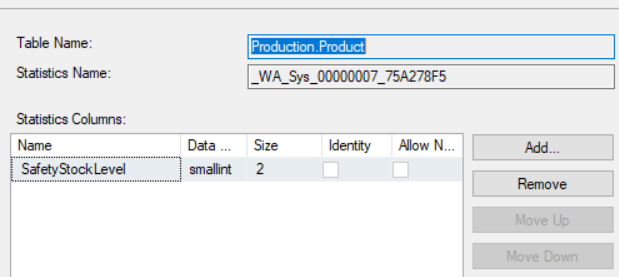Below is a list of the column in the Production.Product table.  Notice that the SafetyStockLevel is the seventh column.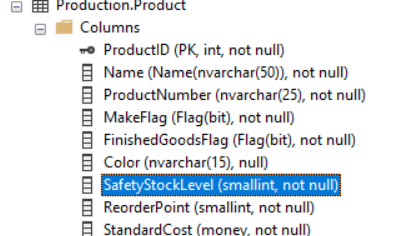But what about this statistics object, _WA_Sys_0000000A_75A278F5.  Where is column A?  The means it is the 10th column as indicated below.  The A means that it is a hexadecimal value.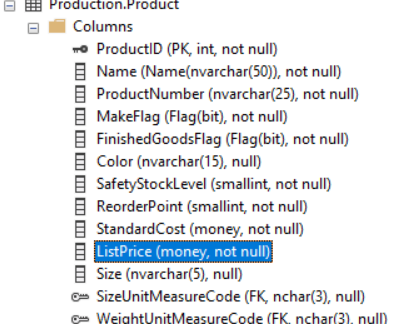That takes care of the first three parts of the name.  The last item, 75A278F5 is a hexadecimal number of the object ID.  The image below you can see that the object ID is the same as 75A278F5 converted to a decimal number.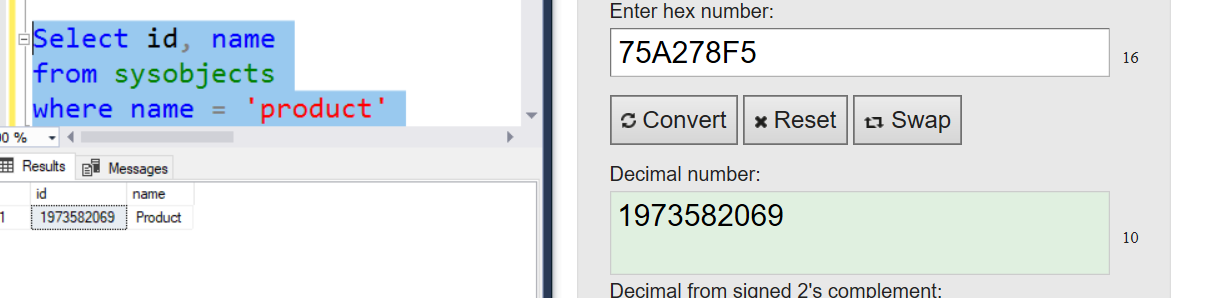Now that we have broken down the name, let’s talk about how these actually get created.

If you run the statement below, it will create the statistics object on the MakeFlag column. Before showing you the results, let’s predict the name.

SELECT Name
, MakeFlag
FROM [Production].[Product]
WHERE Makeflag = 2

We know it will start with _WA_SYS_.  Since MakeFlag is the 4th column, we know the next part of the name is going to be 00000004.  This just leaves the last part, the hexadecimal version of the object id.  Using a converter at https://www.rapidtables.com/convert/number/decimal-to-hex.html.  we can take the object ID and convert it to 75A278F5.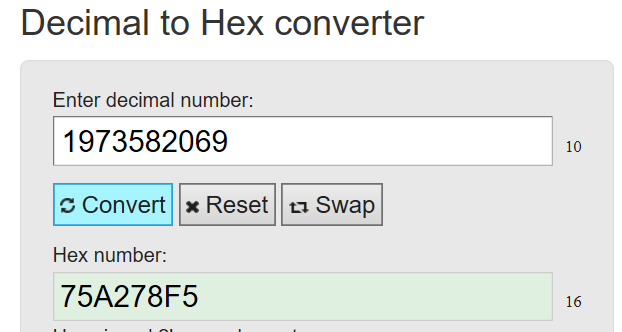Put all the parts together and you get _WA_Sys_00000004_75A278F5 as confirmed by the screenshot below.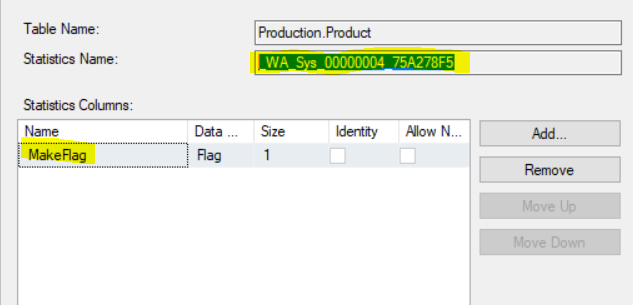After all that, the easiest way to find the system generated statistics is the run this statement:

SELECT *
FROM sys.stats

This statement will return a column named, auto_created.  This is another method of finding the system generated statistics.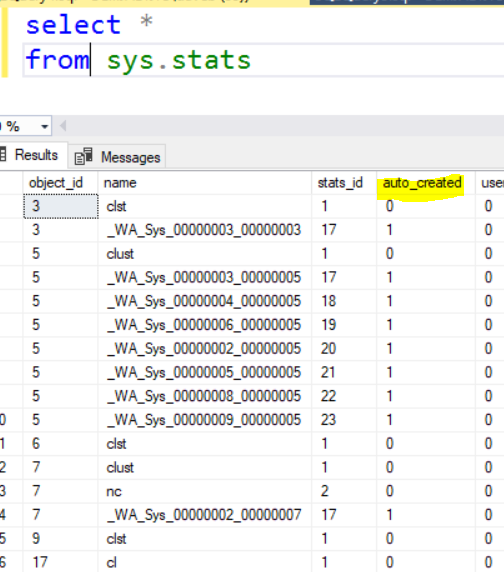Hope this helps clear up the names of system generate statistics.  Thanks for stopping by and hope you learned a little something.